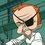# Expected Length of Longest Repeating Substring

This is inspired by one of this week's Data Structures and Algorithms challenges. Suppose you had a string S of length L whose letters are drawn uniformly randomly from an alphabet of size N. A repeating substring is something like 'aaaaaaaa' or '7777' with the same letter appearing consecutively more than once. Let R be the longest repeating substring of S. What is E(length(R))?

Another question that feels related: Suppose we have an ordering on this alphabet. Let's think of the string S as a sequence instead. Call an increasing subsequence one in which letter is less than (or equal) to those that follow it in the subsequence. These need not be consecutive. What is the expected length of the longest increasing subsequence of S?Note by Eric Edwards
7 years ago

This discussion board is a place to discuss our Daily Challenges and the math and science related to those challenges. Explanations are more than just a solution — they should explain the steps and thinking strategies that you used to obtain the solution. Comments should further the discussion of math and science.

When posting on Brilliant:

• Use the emojis to react to an explanation, whether you're congratulating a job well done , or just really confused .
• Ask specific questions about the challenge or the steps in somebody's explanation. Well-posed questions can add a lot to the discussion, but posting "I don't understand!" doesn't help anyone.
• Try to contribute something new to the discussion, whether it is an extension, generalization or other idea related to the challenge.

MarkdownAppears as
*italics* or _italics_ italics
**bold** or __bold__ bold
- bulleted- list
• bulleted
• list
1. numbered2. list
1. numbered
2. list
Note: you must add a full line of space before and after lists for them to show up correctly
paragraph 1paragraph 2

paragraph 1

paragraph 2

[example link](https://brilliant.org)example link
> This is a quote
This is a quote
    # I indented these lines
# 4 spaces, and now they show
# up as a code block.

print "hello world"
# I indented these lines
# 4 spaces, and now they show
# up as a code block.

print "hello world"
MathAppears as
Remember to wrap math in $$ ... $$ or $ ... $ to ensure proper formatting.
2 \times 3 $2 \times 3$
2^{34} $2^{34}$
a_{i-1} $a_{i-1}$
\frac{2}{3} $\frac{2}{3}$
\sqrt{2} $\sqrt{2}$
\sum_{i=1}^3 $\sum_{i=1}^3$
\sin \theta $\sin \theta$
\boxed{123} $\boxed{123}$

Sort by:

The second question seems deeper than I expected. Here's a monograph related to the problem: https://www.math.ucdavis.edu/~romik/lisbook/versions/lis.pdf

The Fields medalist Andrei Okounkov among others did recent-ish work on the fluctuations that relates the longest subsequence of a random permutation (a little different from this problem) to the fluctuations of the largest eigenvalue of a random matrix. Neat.

For my first question, I'll try to code up something for strings of digits in a few bases and see if anything pops out. Meanwhile, if any of you brilliant folks know of an analytic approach, let us know.

- 7 years ago

That's pretty interesting. Maybe you could brute force it for some small numbers and then conjecture something.

- 7 years ago

Quick update: I wrote some code and think I need a faster way to do this. I don't want to put my code here for review because 1) it stinks and isn't commented yet and 2) I don't want to spoil the challenge that inspired this question for anybody. However, I'm getting for random strings that the E(length(R)) is approximately $C\log_N (L)$ with $C$ close to 1.

Can anybody prove it?

- 7 years ago

A very rough estimate for your first question is O(log(L)/log(N)), and for the second one, I believe the answer is O(sqrt(L)), however, here I assumed that the alphabet is of size close to L. If the alphabet is significantly smaller than L, the answer is closer to L/N. I warn you, it could turn out that none of this is correct. I will do some original research and post my results here.

- 7 years ago

The answer for your second question is very close to $2 \sqrt{L}$, assuming the string to be a permutation of the alphabet of size N=L. (Computed for random strings of size up to 20000)

- 7 years ago

There is a wonderful proof of this $2\sqrt{L}$ limit in the first chapter of the book that I linked to which uses the limiting shape of the Young Tableaux arising from applying the RSK correspondence to random permutations.

- 7 years ago

For the first question. If L is fixed then there is a polynomial pL(N) for which E(length(R)) = pL(N)/N^L. The degree of the polynomial will be L. In the case of L = 3, p3(N) = 3*[N] + 2*[2*N*(N-1)] + 1*[N*(N-1)*(N-1)] = (N+2)*N^2. The bracketed terms above count the number of strings with 3, 2, and 1 repeated substrings respectively. Counting the cases becomes increasing complicated, but perhaps a patten will emerge between the polynomials. We know, p1(N) = N and p2(N) = (N+1)*N. I am working on figuring out p4.

- 7 years ago